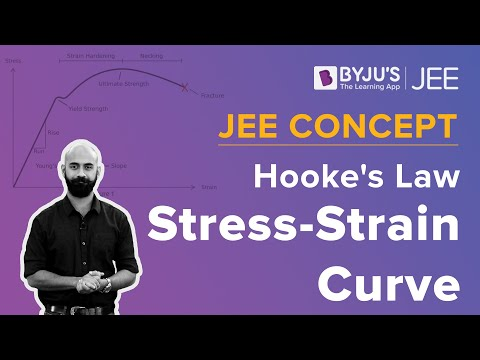# CBSE Class 11 Physics Notes Chapter 9 Mechanical Properties of Solids

According to the CBSE Syllabus 2023-24, this chapter has been renumbered as Chapter 8.

## What is the Elastic Behaviour of Solids?

Elastic Behavior of Solids – What happens to a rubber band when you stretch it and let go? It deforms but regains its original nature when you stop applying a force. But say, you take an aluminium rod and try to bend it using your arm strength. You somehow do manage to bend it a little and then stop applying force. Does the rod regain its original shape? Of course not. It is referred to as the Elastic Behavior of Solids

### What is Elasticity?

This difference in the behaviour of the material is based on their elastic and plastic nature. The rubber band has high elasticity. Elasticity is the ability of a body to resist any permanent changes to it when stress is applied. the body regains its original shape and size when stress application ceases.

### What is the Difference Between Elasticity and Plasticity?

All materials have an elastic limit beyond which, if continuous stress is applied, they will start losing their ability to exhibit perfect elastic behaviour and start deforming. In contrast, plasticity is the non-reversible deformation of solid materials on the application of forces.

Looking at the elasticity at the atomic level, solids are made of atoms (or molecules). They are surrounded by other such atoms which are held in a state of equilibrium by interatomic forces. When an external force is applied these particles are displaced, resulting in the deformation of the solid. When the application of the deforming force is stopped, interatomic forces drive the atoms to regain their state of equilibrium.

The concept of elasticity is an idealization as no material is perfectly elastic. For example, if you use a hair tie to groom yourself, you may have noticed that its size tends to deform after prolonged use. After a point, it may snap as well. This is because the hair tie eventually loses its elastic nature.### Important Points on Elastic Behavior of Solids

• An elastic body is one that regains its original shape and size when deforming forces are removed.
• A plastic body is one that succumbs to deforming forces (however small) and cannot return to its original shape and size.
• Elasticity is the property of a body to regain its original shape and size when deforming forces are removed. It exhibits an opposition to change.
 Also Access NCERT Solutions for Class 11 Physics Chapter 9 NCERT Exemplar for Class 11 Physics Chapter 9

### Important Questions

1. The edge of an aluminium cube is 10 cm long. One face of the cube is firmly fixed to a vertical wall. A mass of 100 kg is then attached to the opposite face of the cube. The shear modulus of aluminium is 25 GPa. What is the vertical deflection of this face?
2. 9.7 Four identical hollow cylindrical columns of mild steel support a big structure of mass 50,000 kg. The inner and outer radii of each column are 30 and 60 cm respectively. Assuming the load distribution to be uniform, calculate the compressional strain of each column.
3. 9.8 A piece of copper having a rectangular cross-section of 15.2 mm × 19.1 mm is pulled in tension with 44,500 N force, producing only elastic deformation. Calculate the resulting strain.

 Bulk Modulus of Elasticity Young’s Modulus and Elastic Modulus

## Frequently Asked Questions on CBSE Class 11 Physics Notes Chapter 9 Mechanical Properties of Solids

Q1

### What is the meaning of elasticity?

The ability of a deformed material body to return to its original shape and size when the forces causing the deformation are removed is known as elasticity.

Q2

### What is the meaning of plasticity?

Plasticity is the deformation of a material undergoing non-reversible changes of shape in response to applied forces.

Q3

### Mention as an example of plastic deformation.

A wet chewing gum is an example of plastic deformation (can be stretched dozens of times to its original length).